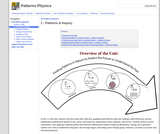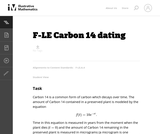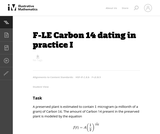Updating search results...

# 48 Results

View
Selected filters:
• LinearConditional Remix & Share Permitted
CC BY-NC-SA
Rating
0.0 stars

In Part 1 of this unit, students will learn about data collection, graphing skills (both by hand and computer aided [Desmos]), and the fundamental mathematical patterns of the course: horizontal line, proportional, linear, quadratic, and inverse. Students perform several experiments, each targeting a different pattern and build the mathematical models of physical phenomena. During each experiment, students start with an uninformed wild guess, then through inquiry and making sense through group consensus, can make an accurate data informed prediction.

Subject:
Physics
Material Type:
Unit of Study
Provider:
Portland Metro STEM Partnership
Provider Set:
Patterns Physics
Date Added:
08/01/2018Conditional Remix & Share Permitted
CC BY-NC-SA
Rating
0.0 stars

A statistics lesson on describing and making claims from data representations, specifically linearly increasing data. Applies ideas of rate-of-change to develop writing a linear equation to fit the data, using the equation to interpolate and extrapolate additional information, and integrating the mathematical interpretation appropriately into a social sciences argument.

Subject:
Mathematics
Material Type:
Lesson Plan
Author:
Sarah Ahmed
Johanna Langill
Date Added:
01/28/2016Unrestricted Use
CC BY
Rating
0.0 stars

This task provides a real world context for interpreting and solving exponential equations. There are two solutions provided for part (a). The first solution demonstrates how to deduce the conclusion by thinking in terms of the functions and their rates of change. The second approach illustrates a rigorous algebraic demonstration that the two populations can never be equal.

Subject:
Mathematics
Functions
Material Type:
Activity/Lab
Provider:
Illustrative Mathematics
Provider Set:
Illustrative Mathematics
Author:
Illustrative Mathematics
Date Added:
05/01/2012Conditional Remix & Share Permitted
CC BY-NC
Rating
0.0 stars

Learners examine the basic types of dimensioning including unidirectional and aligned systems, and linear, aligned, angled, arrowless, chain, datum, chart, tabular, radius, diameter, typical, and reference dimensions.

Subject:
Manufacturing
Material Type:
Interactive
Author:
David Schwid
Francine Nettesheim
Glenn Sokolowski
James Bourassa
John Rosz
Marqulyn Mckinney
Michele Williams
Patrick Hoppe
Steve Whitmoyer
Susan Maloney
Date Added:
06/26/2020Unrestricted Use
CC BY
Rating
0.0 stars

The task requires the student to use logarithms to solve an exponential equation in the realistic context of carbon dating, important in archaeology and geology, among other places. Students should be guided to recognize the use of the natural logarithm when the exponential function has the given base of e, as in this problem. Note that the purpose of this task is algebraic in nature -- closely related tasks exist which approach similar problems from numerical or graphical stances.

Subject:
Mathematics
Functions
Archaeology
Material Type:
Activity/Lab
Provider:
Illustrative Mathematics
Provider Set:
Illustrative Mathematics
Author:
Illustrative Mathematics
Date Added:
05/01/2012Unrestricted Use
CC BY
Rating
0.0 stars

In the task "Carbon 14 Dating'' the amount of Carbon 14 in a preserved plant is studied as time passes after the plant has died. In practice, however, scientists wish to determine when the plant died and, as this task shows, this is not possible with a simple measurement of the amount of Carbon 14 remaining in the preserved plant. The equation for the amount of Carbon 14 remaining in the preserved plant is in many ways simpler here, using 12 as a base.

Subject:
Mathematics
Functions
Material Type:
Activity/Lab
Provider:
Illustrative Mathematics
Provider Set:
Illustrative Mathematics
Author:
Illustrative Mathematics
Date Added:
08/21/2012Unrestricted Use
CC BY
Rating
0.0 stars

This problem introduces the method used by scientists to date certain organic material. It is based not on the amount of the Carbon 14 isotope remaining in the sample but rather on the ratio of Carbon 14 to Carbon 12. This ratio decreases, hypothetically, at a constant exponential rate as soon as the organic material has ceased to absorb Carbon 14, that is, as soon as it dies. This problem is intended for instructional purposes only. It provides an interesting and important example of mathematical modeling with an exponential function.

Subject:
Mathematics
Functions
Material Type:
Activity/Lab
Provider:
Illustrative Mathematics
Provider Set:
Illustrative Mathematics
Author:
Illustrative Mathematics
Date Added:
08/21/2012Unrestricted Use
CC BY
Rating
0.0 stars

This exploratory task requires the student to use a property of exponential functions in order to estimate how much Carbon 14 remains in a preserved plant after different amounts of time.

Subject:
Mathematics
Functions
Material Type:
Activity/Lab
Provider:
Illustrative Mathematics
Provider Set:
Illustrative Mathematics
Author:
Illustrative Mathematics
Date Added:
05/01/2012Unrestricted Use
CC BY
Rating
0.0 stars

This task gives students an opportunity to work with exponential functions in a real world context involving continuously compounded interest. They will study how the base of the exponential function impacts its growth rate and use logarithms to solve exponential equations.

Subject:
Mathematics
Functions
Material Type:
Activity/Lab
Provider:
Illustrative Mathematics
Provider Set:
Illustrative Mathematics
Author:
Illustrative Mathematics
Date Added:
05/01/2012Unrestricted Use
CC BY
Rating
0.0 stars

This module discusses the concept of modeling data with linear functions in Algebra.

Subject:
Algebra
Material Type:
Reading
Provider:
Rice University
Provider Set:
Connexions
Author:
Kenny Felder
Date Added:
02/16/2011Unrestricted Use
CC BY
Rating
0.0 stars

Data structurses

Subject:
Computer Science
Material Type:
Lecture Notes
Author:
aruna r
Date Added:
07/29/2019Unrestricted Use
CC BY
Rating
0.0 stars

This task is designed as a follow-up to the task F-LE Do Two Points Always Determine a Linear Function? Linear equations and linear functions are closely related, and there advantages and disadvantages to viewing a given problem through each of these points of view. This task is intended to show the depth of the standard F-LE.2 and its relationship to other important concepts of the middle school and high school curriculum, including ratio, algebra, and geometry.

Subject:
Mathematics
Functions
Material Type:
Activity/Lab
Provider:
Illustrative Mathematics
Provider Set:
Illustrative Mathematics
Author:
Illustrative Mathematics
Date Added:
05/01/2012Unrestricted Use
CC BY
Rating
0.0 stars

This problem complements the problem ``Do two points always determine a linear function?''

Subject:
Mathematics
Functions
Material Type:
Activity/Lab
Provider:
Illustrative Mathematics
Provider Set:
Illustrative Mathematics
Author:
Illustrative Mathematics
Date Added:
05/01/2012Unrestricted Use
CC BY
Rating
0.0 stars

Elementary Algebra is a work text that covers the traditional topics studied in a modern elementary algebra course. It is intended for students who (1) have no exposure to elementary algebra, (2) have previously had an unpleasant experience with elementary algebra, or (3) need to review algebraic concepts and techniques.

Subject:
Algebra
Material Type:
Full Course
Textbook
Provider:
Rice University
Provider Set:
OpenStax CNX
Author:
Denny Burzynski
Wade Ellis
Date Added:
08/16/2010Unrestricted Use
CC BY
Rating
0.0 stars

An important property of linear functions is that they grow by equal differences over equal intervals. In this task students prove this for equal intervals of length one unit, and note that in this case the equal differences have the same value as the slope. In F.LE Equal Differences over Equal Intervals 2, students prove the property in general (for equal intervals of any length).

Subject:
Mathematics
Functions
Material Type:
Activity/Lab
Provider:
Illustrative Mathematics
Provider Set:
Illustrative Mathematics
Author:
Illustrative Mathematics
Date Added:
05/01/2012Unrestricted Use
CC BY
Rating
0.0 stars

An important property of linear functions is that they grow by equal differences over equal intervals. In this task students prove this for equal intervals of length one unit, and note that in this case the equal differences have the same value as the slope.

Subject:
Mathematics
Functions
Material Type:
Activity/Lab
Provider:
Illustrative Mathematics
Provider Set:
Illustrative Mathematics
Author:
Illustrative Mathematics
Date Added:
05/01/2012Unrestricted Use
CC BY
Rating
0.0 stars

In this task students prove that linear functions grow by equal differences over equal intervals, and that exponential functions grow by equal factors over equal intervals.

Subject:
Mathematics
Functions
Material Type:
Activity/Lab
Provider:
Illustrative Mathematics
Provider Set:
Illustrative Mathematics
Author:
Illustrative Mathematics
Date Added:
05/01/2012Unrestricted Use
CC BY
Rating
0.0 stars

In this task students prove that linear functions grow by equal differences over equal intervals, and that exponential functions grow by equal factors over equal intervals.

Subject:
Mathematics
Functions
Material Type:
Activity/Lab
Provider:
Illustrative Mathematics
Provider Set:
Illustrative Mathematics
Author:
Illustrative Mathematics
Date Added:
05/01/2012Unrestricted Use
CC BY
Rating
4.0 stars

This problem illustrates how an exponentially increasing quantity eventually surpasses a linearly increasing quantity.

Subject:
Mathematics
Functions
Material Type:
Activity/Lab
Provider:
Illustrative Mathematics
Provider Set:
Illustrative Mathematics
Author:
Illustrative Mathematics
Date Added:
05/01/2012Unrestricted Use
CC BY
Rating
0.0 stars

In this task students observe using graphs and tables that a quantity increasing exponentially eventually exceeds a quantity increasing linearly, quadratically, or (more generally) as a polynomial function.

Subject:
Mathematics
Functions
Material Type:
Activity/Lab
Provider:
Illustrative Mathematics
Provider Set:
Illustrative Mathematics
Author:
Illustrative Mathematics
Date Added:
05/01/2012